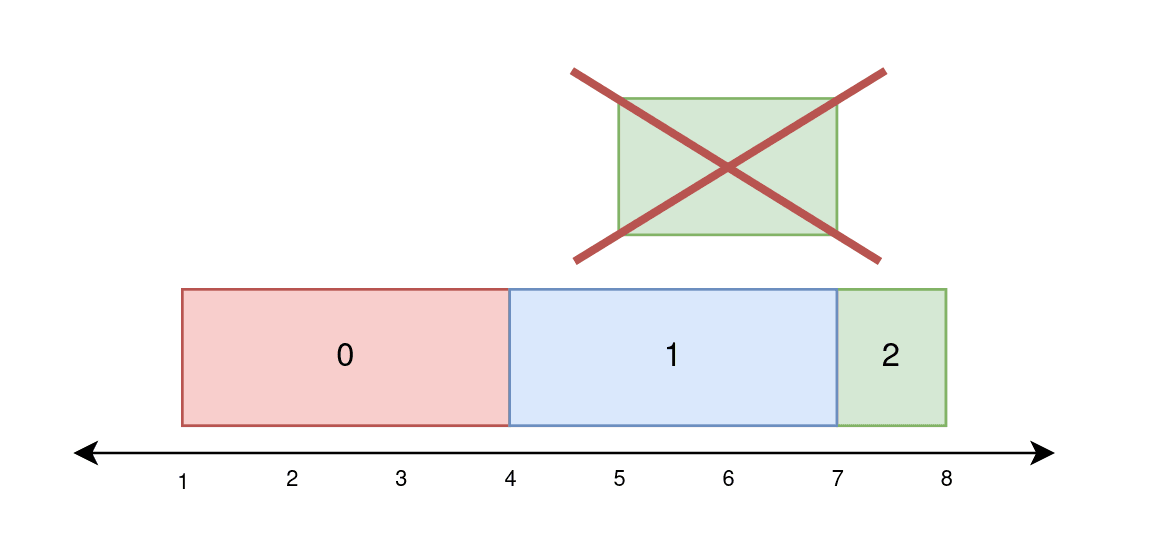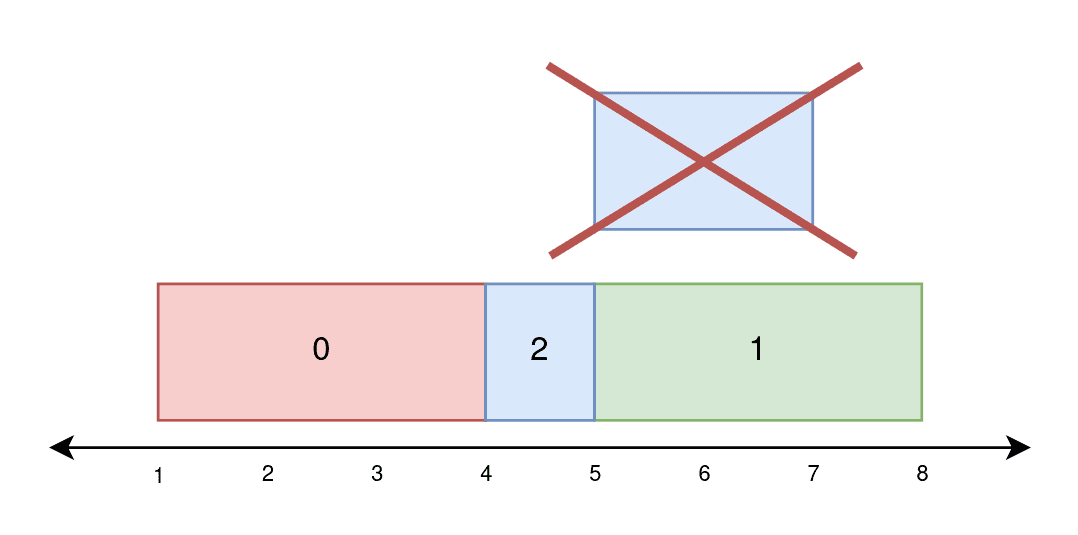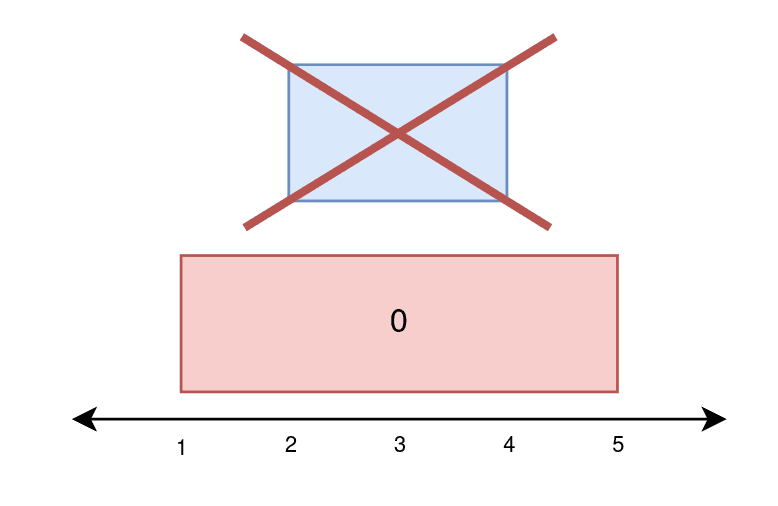##### Welcome to Subscribe On Youtube

Formatted question description: https://leetcode.ca/all/2158.html

# 2158. Amount of New Area Painted Each Day

## Description

There is a long and thin painting that can be represented by a number line. You are given a 0-indexed 2D integer array paint of length n, where paint[i] = [starti, endi]. This means that on the ith day you need to paint the area between starti and endi.

Painting the same area multiple times will create an uneven painting so you only want to paint each area of the painting at most once.

Return an integer array worklog of length n, where worklog[i] is the amount of new area that you painted on the ith day.

Example 1:Input: paint = [[1,4],[4,7],[5,8]]
Output: [3,3,1]
Explanation:
On day 0, paint everything between 1 and 4.
The amount of new area painted on day 0 is 4 - 1 = 3.
On day 1, paint everything between 4 and 7.
The amount of new area painted on day 1 is 7 - 4 = 3.
On day 2, paint everything between 7 and 8.
Everything between 5 and 7 was already painted on day 1.
The amount of new area painted on day 2 is 8 - 7 = 1.


Example 2:Input: paint = [[1,4],[5,8],[4,7]]
Output: [3,3,1]
Explanation:
On day 0, paint everything between 1 and 4.
The amount of new area painted on day 0 is 4 - 1 = 3.
On day 1, paint everything between 5 and 8.
The amount of new area painted on day 1 is 8 - 5 = 3.
On day 2, paint everything between 4 and 5.
Everything between 5 and 7 was already painted on day 1.
The amount of new area painted on day 2 is 5 - 4 = 1.


Example 3:Input: paint = [[1,5],[2,4]]
Output: [4,0]
Explanation:
On day 0, paint everything between 1 and 5.
The amount of new area painted on day 0 is 5 - 1 = 4.
On day 1, paint nothing because everything between 2 and 4 was already painted on day 0.
The amount of new area painted on day 1 is 0.


Constraints:

• 1 <= paint.length <= 105
• paint[i].length == 2
• 0 <= starti < endi <= 5 * 104

## Solutions

Segment Tree.

• class Node {
public:
Node* left;
Node* right;
int l;
int r;
int mid;
int v;

Node(int l, int r) {
this->l = l;
this->r = r;
this->mid = (l + r) >> 1;
this->left = this->right = nullptr;
}
};

class SegmentTree {
private:
Node* root;

public:
SegmentTree() {
root = new Node(1, 100010);
}

void modify(int l, int r, int v) {
modify(l, r, v, root);
}

void modify(int l, int r, int v, Node* node) {
if (l > r) return;
if (node->l >= l && node->r <= r) {
node->v = node->r - node->l + 1;
return;
}
pushdown(node);
if (l <= node->mid) modify(l, r, v, node->left);
if (r > node->mid) modify(l, r, v, node->right);
pushup(node);
}

int query(int l, int r) {
return query(l, r, root);
}

int query(int l, int r, Node* node) {
if (l > r) return 0;
if (node->l >= l && node->r <= r) return node->v;
pushdown(node);
int v = 0;
if (l <= node->mid) v += query(l, r, node->left);
if (r > node->mid) v += query(l, r, node->right);
return v;
}

void pushup(Node* node) {
node->v = node->left->v + node->right->v;
}

void pushdown(Node* node) {
if (!node->left) node->left = new Node(node->l, node->mid);
if (!node->right) node->right = new Node(node->mid + 1, node->r);
Node* left = node->left;
Node* right = node->right;
left->v = left->r - left->l + 1;
right->v = right->r - right->l + 1;
}
}
};

class Solution {
public:
vector<int> amountPainted(vector<vector<int>>& paint) {
int n = paint.size();
vector<int> ans(n);
SegmentTree* tree = new SegmentTree();
for (int i = 0; i < n; ++i) {
int l = paint[i] + 1;
int r = paint[i];
int v = tree->query(l, r);
ans[i] = r - l + 1 - v;
tree->modify(l, r, 1);
}
return ans;
}
};


• class Node:
def __init__(self, l, r):
self.left = None
self.right = None
self.l = l
self.r = r
self.mid = (l + r) >> 1
self.v = 0

class SegmentTree:
def __init__(self):
self.root = Node(1, 10**5 + 10)

def modify(self, l, r, v, node=None):
if l > r:
return
if node is None:
node = self.root
if node.l >= l and node.r <= r:
node.v = node.r - node.l + 1
return
self.pushdown(node)
if l <= node.mid:
self.modify(l, r, v, node.left)
if r > node.mid:
self.modify(l, r, v, node.right)
self.pushup(node)

def query(self, l, r, node=None):
if l > r:
return 0
if node is None:
node = self.root
if node.l >= l and node.r <= r:
return node.v
self.pushdown(node)
v = 0
if l <= node.mid:
v += self.query(l, r, node.left)
if r > node.mid:
v += self.query(l, r, node.right)
return v

def pushup(self, node):
node.v = node.left.v + node.right.v

def pushdown(self, node):
if node.left is None:
node.left = Node(node.l, node.mid)
if node.right is None:
node.right = Node(node.mid + 1, node.r)
left, right = node.left, node.right
left.v = left.r - left.l + 1
right.v = right.r - right.l + 1

class Solution:
def amountPainted(self, paint: List[List[int]]) -> List[int]:
tree = SegmentTree()
ans = []
for i, (start, end) in enumerate(paint):
l, r = start + 1, end
v = tree.query(l, r)
ans.append(r - l + 1 - v)
tree.modify(l, r, 1)
return ans